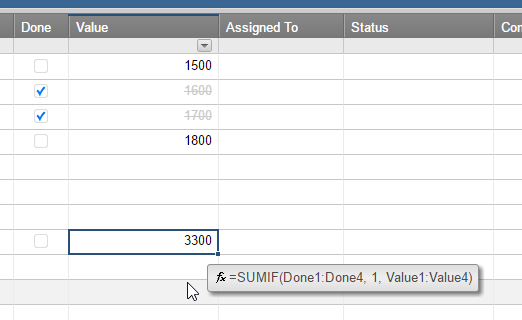#### Welcome to the Smartsheet Forum Archives

The posts in this forum are no longer monitored for accuracy and their content may no longer be current. If there's a discussion here that interests you and you'd like to find (or create) a more current version, please Visit the Current Forums.

# Totaling a column with check boxes

I couldn't find an answer that solved my problem so am asking this question. I am trying to sum up a column where some of the fields are checked and some are not. Can you please help me? I have tried looking at the SUMIF calculations and keep getting the #BOOLEAN EXPECTED error.

Linda

Tags:

• Try the =COUNTIF Formula

Something like

=COUNTIF(RangeOfCellsToCount(CheckboxColumnName), 1)

• Is this what you need?• Sorry! I overlooked the "Sum up a column" request, since I've been working on a sheet with counting checked boxes!

• Adam Overton in your example, I would only be adding the check box column, not any other columns. I have a column of 40 (approx) lines and I need a total of who's qualified by a checked box. Does that make sense? Thank you.

• Linda, I'm sorry, I'm not understanding. You can see in my example that the total is 3300, because that is the sum of the checked items (1600+1700). How is that different than what you are asking?

• Linda,

Are you looking for a SUM of CHECKED BOXES?

OR a SUM of VALUES that are CHECKED?

• Hi Linda,

Disclaimers:

- You can't place these formulas in the checkbox column, as checkbox columns will only work with formulas that return a boolean value (0 or 1 in Smartsheet) and will give you an error otherwise.

- You'll need to modify the following examples to reference columns in your sheet.

If you need to COUNT the number of checked boxes, use a formula similar to this example:

=COUNTIF(Done:Done, 1)

If you need to SUM values in one column IF a corresponding checkbox is checked, use this type of formula:

=SUMIF(Done:Done, 1, Value:Value)

• Shaine that makes perfect sense. Thank you, I'll try adding them in a non-checked column.

• One clarification to Shaine's point.

You CAN put the calculation into the CheckBox column, but the result must be converted to text by adding

+ ""

to it.

If the count or sum then needs to be used in another formula, it must be converted to a number in that formula using the VALUE() function.

=SUMIF(Done:Done, 1, Value:Value) + ""

may return something that looks like 23, but it is text 23, not number 23.

1 + text 23 is 123, not 24.

If there are a lot of rows in the sheet, then adding a column for one (or a small number) of calculations may cause other problems.

Craig

This discussion has been closed.